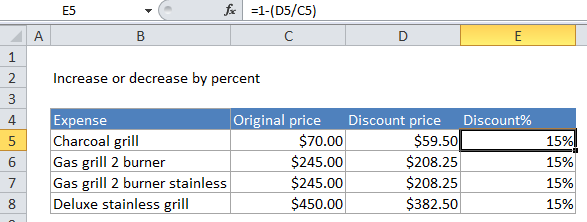## Excel Office

Excel How Tos, Tutorials, Tips & Tricks, Shortcuts

# How to calculate percentage discount in Excel

If you have a discounted price and an original price, and you want to know the discount as a percentage, you can calculate the percentage discount using a formula that divides the discounted price by the original price and then subtracts the result from one.

Worked Example:   How to calculate total from percentage in Excel

## Formula

`= 1-(discount_price/original_price)`